# Passing Division Program in Java

In this tutorial you will learn about the Passing Division Program in Java and its application with practical example.

In this tutorial, we will learn to create a Java to Program Find Passing Division Program in Java using java programming.

## Prerequisites

Before starting with this tutorial, we assume that you are best aware of the following Java programming concepts:

• Java Operators.
• Basic Input and Output function in Java.
• Class and Object in Java.
• Basic Java programming.
• If-else statements in Java.
• For loop in Java.

## How to Calculate percentage?

Percent is one out of one hundred parts, i.e., A ratio of the parts out of 100. Symbol of a percent is “%”.

Example :->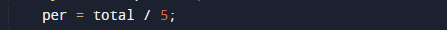## Passing Division Program in Java

In this program we will find Passing Division number provided by user. first will take the input from the user and then calculate passing percentage of a user. Lets have look at the Code.

## Output

Division:=>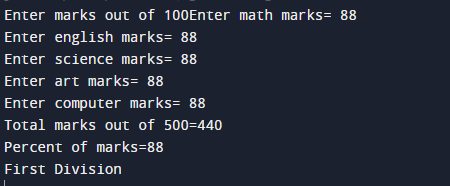In the above program, we have first declared and initialized a set variables required in the program.

• m = it will hold entered math’s number.
• e = it will hold entered English number.
• s = it will hold entered science number.
• art = it will hold entered art  number.
• com = it will hold entered computer number.
• total= it will hold total of all subject.
• per =it will hold percentage  of a student.

After that we take 5 subjects values form user.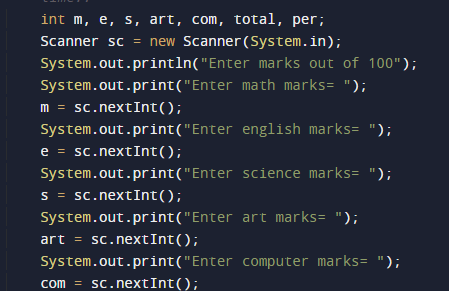and then after that we add all values in variable total and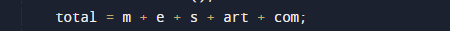then after find percentageand finally we will print the percentage according to inputted values.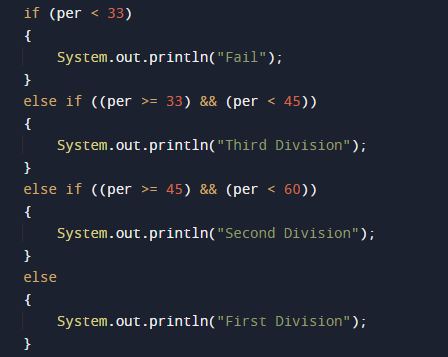In this tutorial we have learn about the Passing Division Program in Java and its application with practical example. I hope you will like this tutorial.